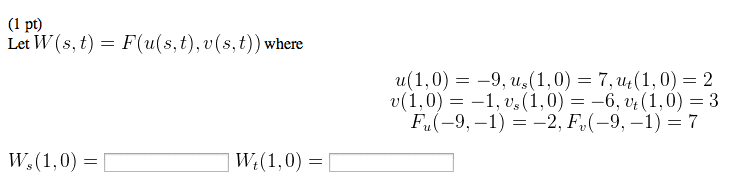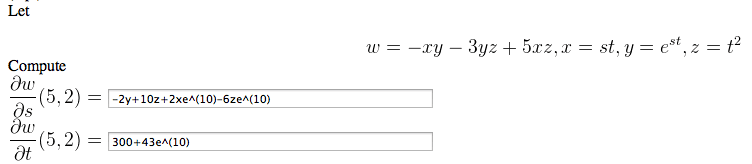1
0
watching
40
views

# 1.Let W(s, t) = F(u(s, t), v(s, t)) where u(1, 0) = -9, us(1, 0) = 7, ut(1,0) = 2 v(1, 0) = -1, vs(1, 0) = -6, vt(1, 0) = 3 Fu(-9, -1) = -2, Fv(-9, -1) = 7 Ws(1, 0) = Wt(1, 0) = Let w = -xy - 3yz + 5 xz, x = st, y = est, z = t2 Compute (5, 2) = -2 + 10z + 2xe^(10) - 6ze^(10) (5, 2) = 300 + 43e^(10) Show transcribed image text Let W(s, t) = F(u(s, t), v(s, t)) where u(1, 0) = -9, us(1, 0) = 7, ut(1,0) = 2 v(1, 0) = -1, vs(1, 0) = -6, vt(1, 0) = 3 Fu(-9, -1) = -2, Fv(-9, -1) = 7 Ws(1, 0) = Wt(1, 0) = Let w = -xy - 3yz + 5 xz, x = st, y = est, z = t2 Compute (5, 2) = -2 + 10z + 2xe^(10) - 6ze^(10) (5, 2) = 300 + 43e^(10)